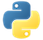# CombinePerKeyPydoc

Combines all elements for each key in a collection.

## Examples

In the following examples, we create a pipeline with a `PCollection` of produce. Then, we apply `CombinePerKey` in multiple ways to combine all the elements in the `PCollection`.

`CombinePerKey` accepts a function that takes a list of values as an input, and combines them for each key.

### Example 1: Combining with a predefined function

We use the function `sum` which takes an `iterable` of numbers and adds them together.

``````import apache_beam as beam

with beam.Pipeline() as pipeline:
total = (
pipeline
| 'Create plant counts' >> beam.Create([
('🥕', 3),
('🥕', 2),
('🍆', 1),
('🍅', 4),
('🍅', 5),
('🍅', 3),
])
| 'Sum' >> beam.CombinePerKey(sum)
| beam.Map(print))``````

Output:

``````('🥕', 5)
('🍆', 1)
('🍅', 12)``````View source code

### Example 2: Combining with a function

We define a function `saturated_sum` which takes an `iterable` of numbers and adds them together, up to a predefined maximum number.

``````import apache_beam as beam

def saturated_sum(values):
max_value = 8
return min(sum(values), max_value)

with beam.Pipeline() as pipeline:
saturated_total = (
pipeline
| 'Create plant counts' >> beam.Create([
('🥕', 3),
('🥕', 2),
('🍆', 1),
('🍅', 4),
('🍅', 5),
('🍅', 3),
])
| 'Saturated sum' >> beam.CombinePerKey(saturated_sum)
| beam.Map(print))``````

Output:

``````('🥕', 5)
('🍆', 1)
('🍅', 8)``````View source code

### Example 3: Combining with a lambda function

We can also use lambda functions to simplify Example 2.

``````import apache_beam as beam

with beam.Pipeline() as pipeline:
saturated_total = (
pipeline
| 'Create plant counts' >> beam.Create([
('🥕', 3),
('🥕', 2),
('🍆', 1),
('🍅', 4),
('🍅', 5),
('🍅', 3),
])
| 'Saturated sum' >>
beam.CombinePerKey(lambda values: min(sum(values), 8))
| beam.Map(print))``````

Output:

``````('🥕', 5)
('🍆', 1)
('🍅', 8)``````View source code

### Example 4: Combining with multiple arguments

You can pass functions with multiple arguments to `CombinePerKey`. They are passed as additional positional arguments or keyword arguments to the function.

In this example, the lambda function takes `values` and `max_value` as arguments.

``````import apache_beam as beam

with beam.Pipeline() as pipeline:
saturated_total = (
pipeline
| 'Create plant counts' >> beam.Create([
('🥕', 3),
('🥕', 2),
('🍆', 1),
('🍅', 4),
('🍅', 5),
('🍅', 3),
])
| 'Saturated sum' >> beam.CombinePerKey(
lambda values, max_value: min(sum(values), max_value), max_value=8)
| beam.Map(print))``````

Output:

``````('🥕', 5)
('🍆', 1)
('🍅', 8)``````View source code

### Example 5: Combining with side inputs as singletons

If the `PCollection` has a single value, such as the average from another computation, passing the `PCollection` as a singleton accesses that value.

In this example, we pass a `PCollection` the value `8` as a singleton. We then use that value as the `max_value` for our saturated sum.

``````import apache_beam as beam

with beam.Pipeline() as pipeline:
max_value = pipeline | 'Create max_value' >> beam.Create()

saturated_total = (
pipeline
| 'Create plant counts' >> beam.Create([
('🥕', 3),
('🥕', 2),
('🍆', 1),
('🍅', 4),
('🍅', 5),
('🍅', 3),
])
| 'Saturated sum' >> beam.CombinePerKey(
lambda values,
max_value: min(sum(values), max_value),
max_value=beam.pvalue.AsSingleton(max_value))
| beam.Map(print))``````

Output:

``````('🥕', 5)
('🍆', 1)
('🍅', 8)``````View source code

### Example 6: Combining with side inputs as iterators

If the `PCollection` has multiple values, pass the `PCollection` as an iterator. This accesses elements lazily as they are needed, so it is possible to iterate over large `PCollection`s that won’t fit into memory.

``````import apache_beam as beam

def bounded_sum(values, data_range):
min_value = min(data_range)
result = sum(values)
if result < min_value:
return min_value
max_value = max(data_range)
if result > max_value:
return max_value
return result

with beam.Pipeline() as pipeline:
data_range = pipeline | 'Create data_range' >> beam.Create([2, 4, 8])

bounded_total = (
pipeline
| 'Create plant counts' >> beam.Create([
('🥕', 3),
('🥕', 2),
('🍆', 1),
('🍅', 4),
('🍅', 5),
('🍅', 3),
])
| 'Bounded sum' >> beam.CombinePerKey(
bounded_sum, data_range=beam.pvalue.AsIter(data_range))
| beam.Map(print))``````

Output:

``````('🥕', 5)
('🍆', 2)
('🍅', 8)``````View source code

Note: You can pass the `PCollection` as a list with `beam.pvalue.AsList(pcollection)`, but this requires that all the elements fit into memory.

### Example 7: Combining with side inputs as dictionaries

If a `PCollection` is small enough to fit into memory, then that `PCollection` can be passed as a dictionary. Each element must be a `(key, value)` pair. Note that all the elements of the `PCollection` must fit into memory for this. If the `PCollection` won’t fit into memory, use `beam.pvalue.AsIter(pcollection)` instead.

``````import apache_beam as beam

def bounded_sum(values, data_range):
min_value = data_range['min']
result = sum(values)
if result < min_value:
return min_value
max_value = data_range['max']
if result > max_value:
return max_value
return result

with beam.Pipeline() as pipeline:
data_range = pipeline | 'Create data_range' >> beam.Create([
('min', 2),
('max', 8),
])

bounded_total = (
pipeline
| 'Create plant counts' >> beam.Create([
('🥕', 3),
('🥕', 2),
('🍆', 1),
('🍅', 4),
('🍅', 5),
('🍅', 3),
])
| 'Bounded sum' >> beam.CombinePerKey(
bounded_sum, data_range=beam.pvalue.AsDict(data_range))
| beam.Map(print))``````

Output:

``````('🥕', 5)
('🍆', 2)
('🍅', 8)``````View source code

### Example 8: Combining with a `CombineFn`

The more general way to combine elements, and the most flexible, is with a class that inherits from `CombineFn`.

``````import apache_beam as beam

class AverageFn(beam.CombineFn):
def create_accumulator(self):
sum = 0.0
count = 0
accumulator = sum, count
return accumulator

sum, count = accumulator
return sum + input, count + 1

def merge_accumulators(self, accumulators):
# accumulators = [(sum1, count1), (sum2, count2), (sum3, count3), ...]
sums, counts = zip(*accumulators)
# sums = [sum1, sum2, sum3, ...]
# counts = [count1, count2, count3, ...]
return sum(sums), sum(counts)

def extract_output(self, accumulator):
sum, count = accumulator
if count == 0:
return float('NaN')
return sum / count

with beam.Pipeline() as pipeline:
average = (
pipeline
| 'Create plant counts' >> beam.Create([
('🥕', 3),
('🥕', 2),
('🍆', 1),
('🍅', 4),
('🍅', 5),
('🍅', 3),
])
| 'Average' >> beam.CombinePerKey(AverageFn())
| beam.Map(print))``````

Output:

``````('🥕', 2.5)
('🍆', 1.0)
('🍅', 4.0)``````View source code

You can use the following combiner transforms:

See also GroupBy which allows you to combine more than one field at once.Pydoc